# Modeled interventions in mrgsolve

This post is to introduce modeled interventions in mrgsolve. The main use case is to force mrgsolve to advance the system to a specific time so that some aspect of the system can change at that time.

modeled events
mtime
Author

Kyle Baron

Published

January 1, 2018

# 1 Introduction

This post is to introduce modeled interventions in mrgsolve. The main use case is to force mrgsolve to advance the system to a specific time so that some aspect of the system can change at that time. This is similar to the `MTIME` functionality that NONMEM provides. Doses (bolus or infusions) can also be implemented as a modeled event. These events are “modeled” because they originate from code in the `\$MAIN` block, not the input data set, so that the events can be reactive to model parameters or the state of the model itself.

# 2 Status

At the time of this post, this functionality is only in a development branch on the mrgsolve GitHub. But we’ve been working on and revising this concept for a while now, so hopefully it is more or less stable with a reasonable interface. That said, specifics or syntax may change before this gets rolled out to CRAN.

# 3 Example: time-varying KA

One common use case for modeled events are a time-varying model parameter such as an absorption rate constant. The model with constant `KA` looks like this

``````code <- '
\$PARAM CL = 1, V = 20, KA1 = 0.1, KA2 = 0.5, KA3 = 5

\$PKMODEL cmt = "GUT CENT", depot = TRUE

\$PREAMBLE capture KA = KA1;

\$MAIN
KA = KA2;
' ``````
``````library(mrgsolve)

mcode_cache("mevent0", code) %>%
ev(amt = 100) %>%
mrgsim(end = 24, delta = 0.1) %>%
plot(CENT+KA~time)``````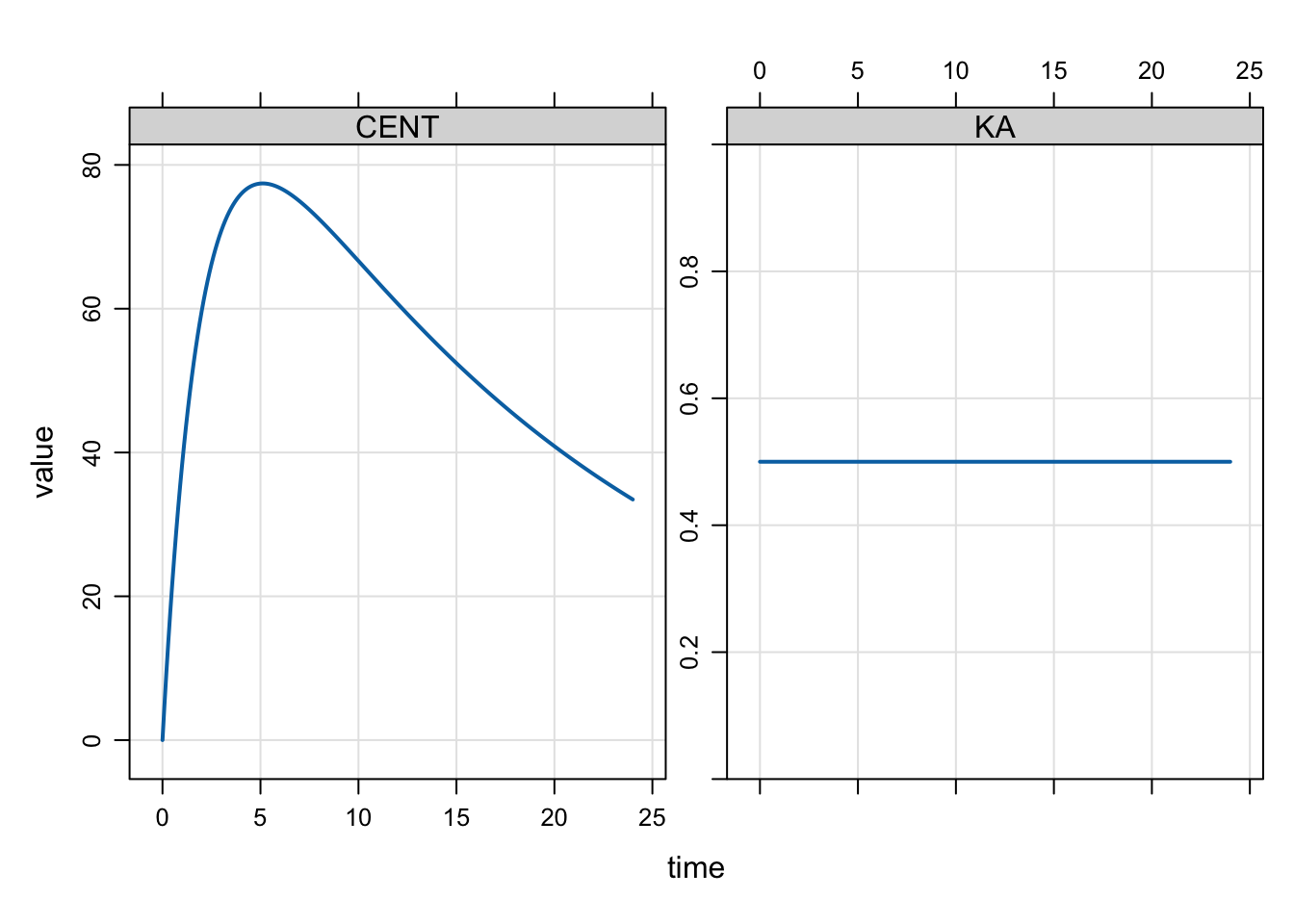For the model with time-varying parameter, we want `KA` to start with a lower value at the time of the oral dose and then increase at some time after the dose. And we want that to happen for every dose. We model the time after the dose when we want KA to change and force the simulation to stop at that time so we can make the required changes.

The model with time-varying `KA` might look like this

``````code <- '
\$PARAM CL = 1, V = 20, KA1 = 0.1, KA2 = 0.5, KA3 = 5

\$PKMODEL cmt = "GUT CENT", depot = TRUE

\$MAIN

if(NEWIND <=1) capture KA = KA1;

if(EVID==1) {
KA = KA1;
self.mevent(TIME + 2, 33);
}

if(EVID==33) KA = KA3;

' ``````

The arguments for `self.mevent()` in this case are:

1. time
2. evid

Whenever we see a dose (`EVID==1`), we set `KA` to the initial (slower) `KA` value and register a model event that in this case happens two hours later. We use `EVID=33` for that change point event. This is a arbitrary choice for `EVID` (it could be [almost] anything). The idea here is that, when we see `EVID==33` come along, we change the `KA` to the faster value.

We don’t have plans to offer indicators like `MPAST` or `MTDIFF` as NONMEM does. But we can achieve similar behavior by tagging the event and then looking for that tag again down the road.

The simulation looks like this

``mod <- mcode_cache("mevent", code)``
``Building mevent ... done.``
``````mod %>%
ev(amt = 100) %>%
mrgsim(end = 24, delta = 0.1) %>%
plot(CENT+KA~time)``````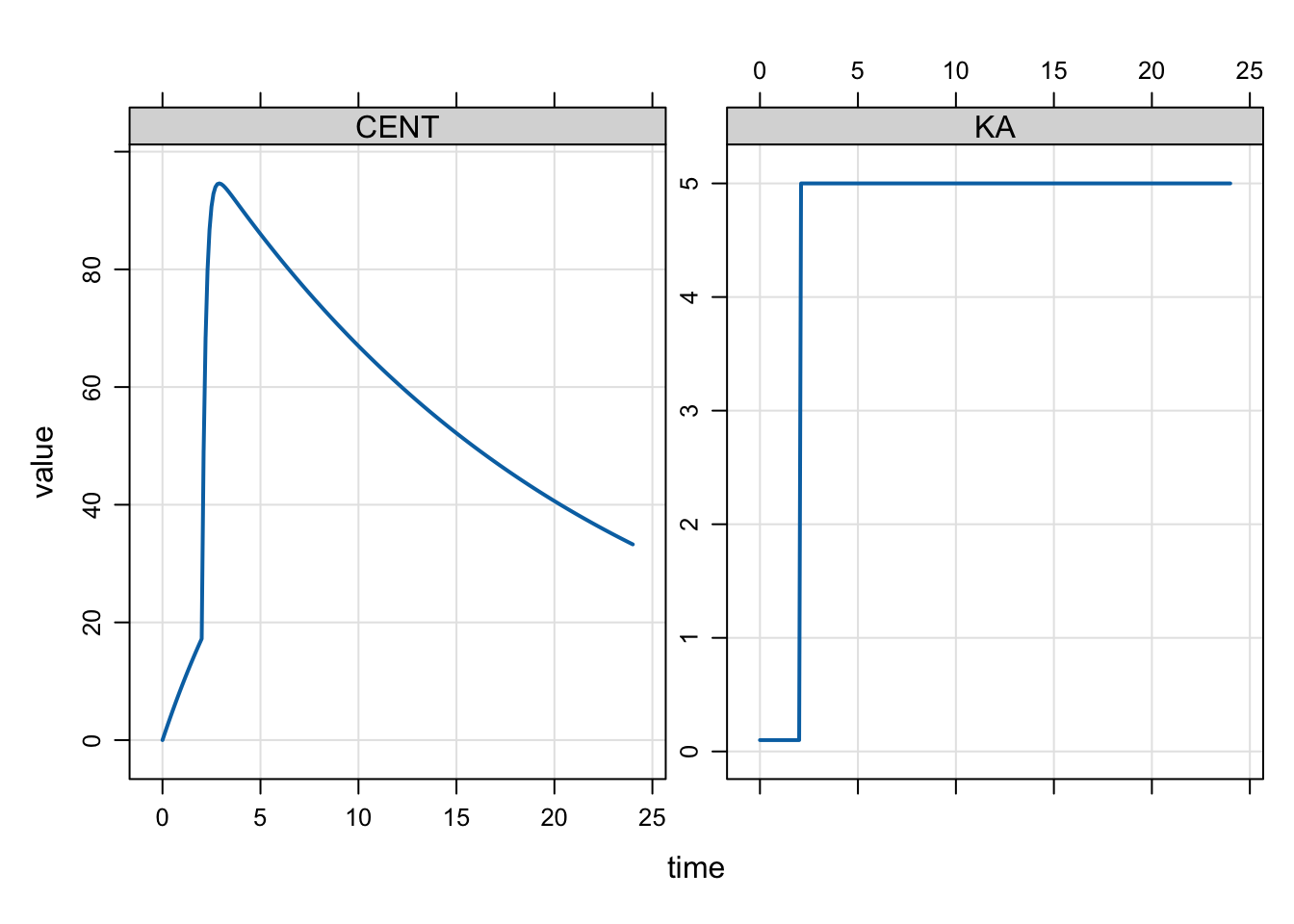We can refine this even more with an additional intervention time for an intermediate value so that `KA` changes twice as the dose is absorbed

``````code <- '
\$PARAM CL = 1, V = 20, KA1 = 0.1, KA2 = 0.5, KA3 = 5

\$PKMODEL cmt = "GUT CENT", depot = TRUE

\$PREAMBLE capture KA = KA1;

\$MAIN

if(EVID==1) {
KA = KA1;
self.mevent(TIME + 1, 33);
self.mevent(TIME + 2, 34);
}

if(EVID==33) KA = KA2;
if(EVID==34) KA = KA3;

' ``````

We use a separate `EVID` (34) here to distinguish the second change from the first change (with `EVID 33`).

``mod <- mcode_cache("mevent2", code)``
``Building mevent2 ... done.``
``````mod %>%
ev(amt = 100) %>%
mrgsim(end = 8, delta = 0.1) %>%
plot(CENT+KA~time)``````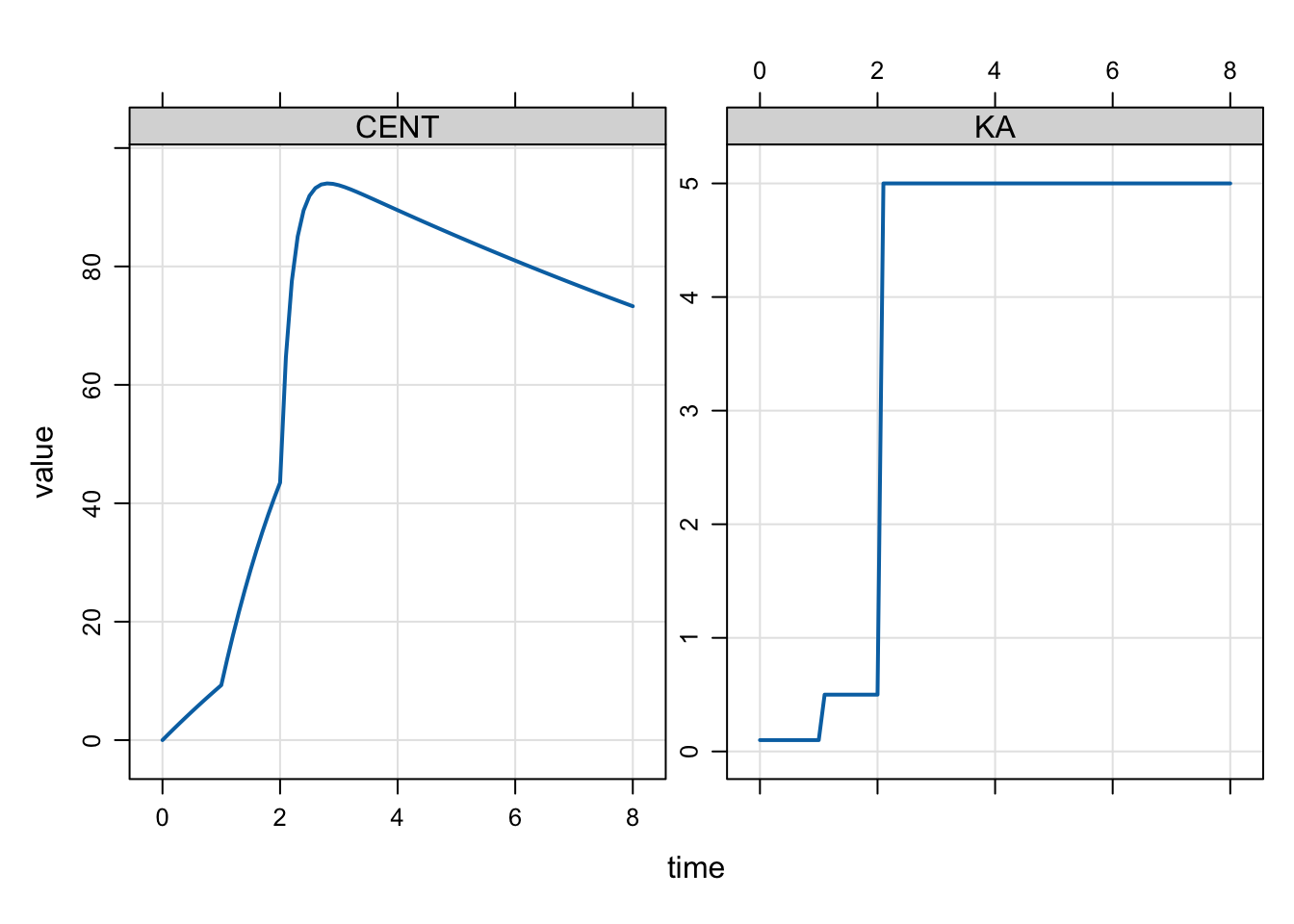Now we have the two change points implemented.

Multiple doses would look like this

``````mod %>%
ev(amt = 100, ii = 24, addl = 2) %>%
mrgsim(end = 120, delta = 0.1) %>%
plot(CENT+KA~time)``````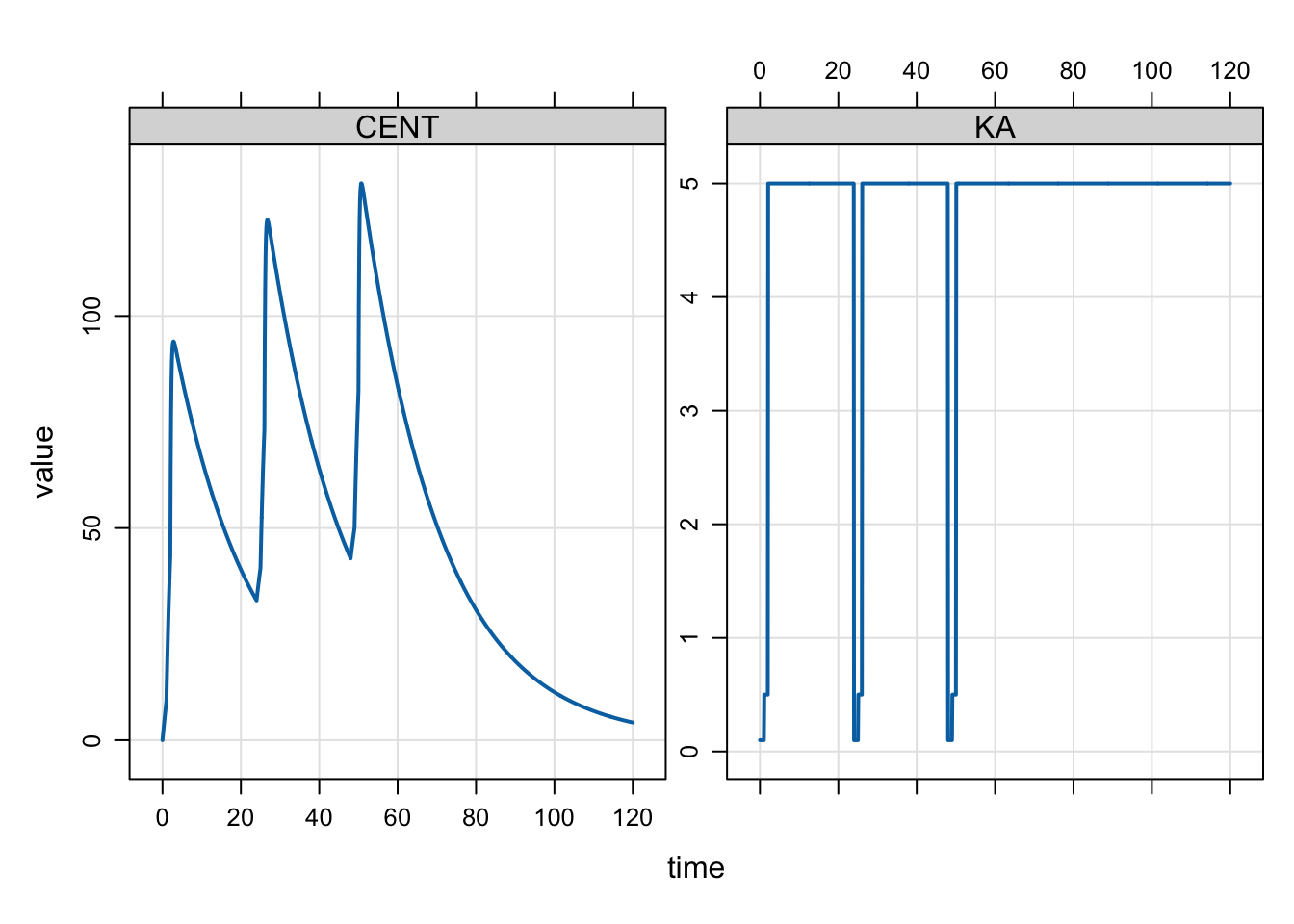And we can model this so that the change point is a function of a model parameter

``````code <- '
\$PARAM CL = 1, V = 20, KA1 = 0.1, KA2 = 0.5, KA3 = 5
MTIME1 = 2

\$PKMODEL cmt = "GUT CENT", depot = TRUE

\$PREAMBLE capture KA = KA1;

\$MAIN

if(EVID==1) {
KA = KA1;
self.mevent(TIME + MTIME1, 33);
}

if(EVID==33) KA = KA3;

' ``````
``mod <- mcode_cache("mevent3", code)``
``Building mevent3 ... done.``
``````idata <- data.frame(MTIME1 = seq(0,2.5,0.5))

mod %>%
ev(amt = 100) %>%
idata_set(idata) %>%
mrgsim(end = 8, delta = 0.1) %>%
plot(CENT+KA~time)``````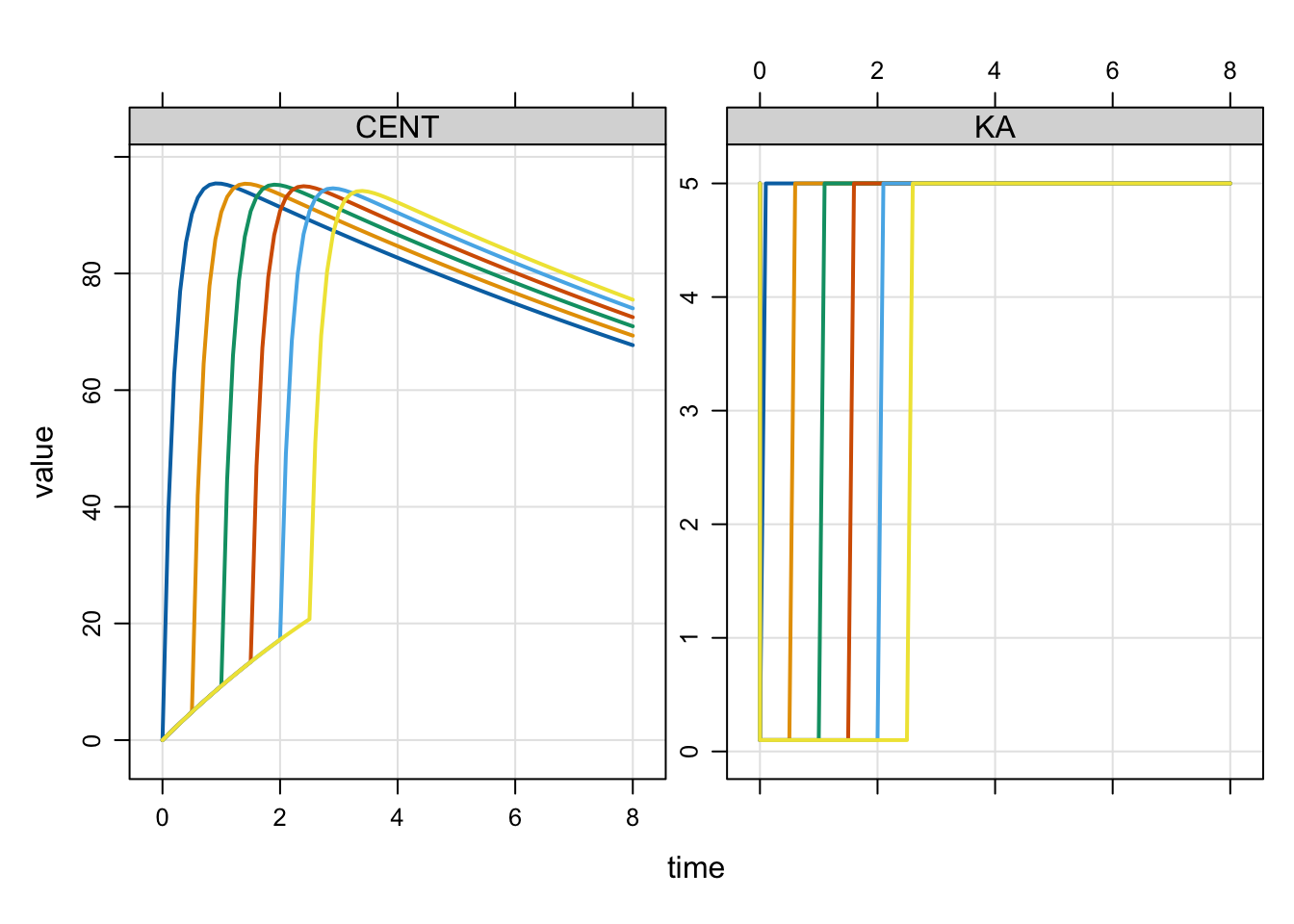# 4 Example: modeled dosing events

In the previous example, we used the `mevent` call to to force the simulation to stop at the time we wanted a model parameter to change. This was accomplished by an event object with `EVID=2`, which forces the solver to stop, reset, and then keep going with the new model parameters.

We can also use this event mechanism to implement actual doses. Now at the change point, we add some mass to one of the compartments. An example model for q12 hour dosing might look like:

``````code <- '
\$PARAM CL = 1, V = 20, KA = 2

\$PKMODEL cmt = "GUT CENT", depot = TRUE

\$MAIN

if(TIME/12 ==floor(TIME/12) && TIME < 120) {
mrg::evdata ev(TIME,1);
ev.cmt = 1;
ev.amt = 100;
self.mevector.push_back(ev);
}

' ``````
``mod <- mcode_cache("mevent4", code)``
``Building mevent4 ... done.``
``mod %>%  mrgsim(end = 240, delta = 0.2) %>% plot(CENT~time)``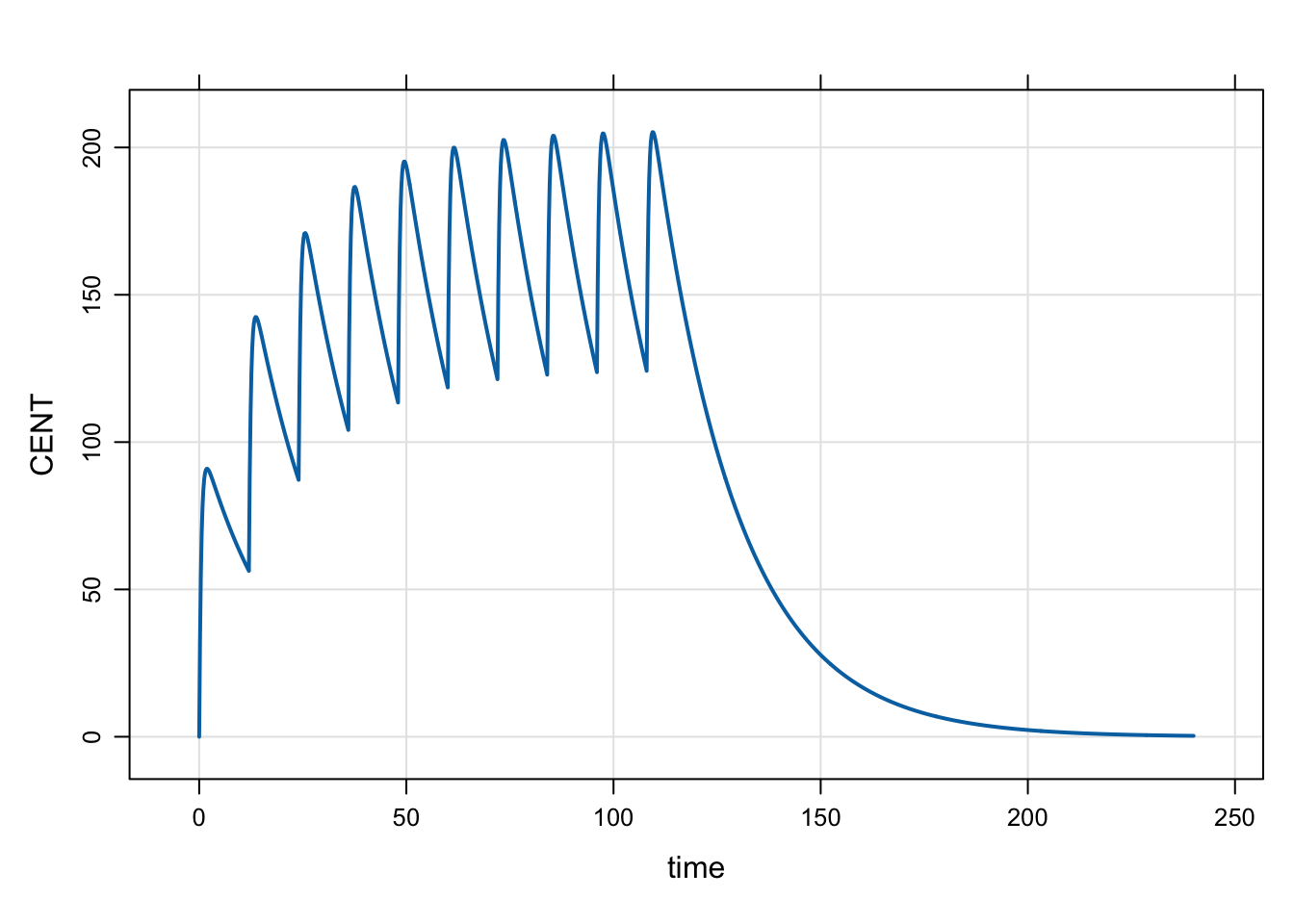So here, we simulated without the data set and implemented the dosing from within the model. This isn’t the way you should be implementing dosing like this on a regular basis, but it shows how you can trigger doses from within the model based on what has gone on up to that point in the simulation.